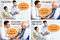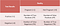# Statistical Performance Measures

How to select the best model from the available options?

Statistical performance measures are often used for model selection in machine learning and statistical inference. From multiple models trained with different sets of hyper-parameters and parameters, the one that gives best performance in terms of a selected performance criterion is finally adopted. Let’s understand commonly used performance metrics for model selection through an example so that we can choose one of these for our model selection.

Let’s assume that we have a pregnancy test that gives us binary results, positive (or 1), if tested individual is pregnant and negative (or 0) if tested individual is not pregnant (~pregnant). Now, we can define the following outputs for this binary test-

True positive: Test results are + when an individual is pregnant

True negative: Test results are - when an individual is not pregnant

False positive (Type I error): Test results are + when an individual is not pregnant

False negative (Type II error): Test results are - when an individual is pregnant

These four scenarios are illustrated in the following figure.Figure 1 Four possible combinations of reality and our binary pregnancy test results

Let’s say we evaluate N randomly selected individuals for pregnancy using our test and note down the number of True positives, False positives, True negatives and False negatives. Using these numbers we can create a 2 x 2 matrix, known as confusion matrix, as shown below-Figure 2 Confusion matrix for comparing reality (or ground-truth) with the binary pregnancy test results

Using information in Figure 2, we can define the following performance metrics to assess the quality of our pregnancy test-

Sensitivity (recall or true positive rate, TPR): Divide the number of true positives (test results) with total number (out of N) of pregnant women (ground truth) to compute sensitivity as follows:

Sensitivity or recall gives us the conditional probability of getting a + test result given that the individual is pregnant, p(test = + | individual = pregnant). Sensitivity (or recall) is a good measure for model selection if the cost of false negatives is very high. For our pregnancy test, the cost of a false negative decision (test negative for an actually pregnant individual) is indeed very high compared to that of a false positive decision (test positive for an individual not pregnant) because of the healthcare issues involved.

Specificity (true negative rate, TNR): Divide the number of true negatives (test results) with total number (out of N) of women who are not pregnant (ground truth) to compute sensitivity as follows:

Specificity gives us the conditional probability of getting a - test result given that the individual is not pregnant, p(test = - | individual = ~pregnant).
Specificity assesses the test’s ability to correctly identify patients who are not pregnant.

Precision (positive predictive value, PPV): Divide the number of true positives (test results) with the total number of predicted positives (test results) to compute precision as follows:

Precision is a good measure for model selection if the cost of false positives is very high. Notice the difference between sensitivity and precision, sensitivity is computed with respect to the ground truth while precision uses only test results in its computation.

False discovery rate (FDR): It simply tells us how many false positives are given by our pregnancy test out of the total number of predicted positives (test results) and can be computed as follows:

Negative predictive value (NPV): Divide the number of true negatives (test results) with the total number of predicted negatives (test results) as follows:

Notice the difference between specificity and NPV, specificity is computed with respect to the ground truth while NPV uses only test results.

The aforementioned measures mostly emphasize on one type of model errors or correctness as indicated by terms in their numerators and denominators. For example, sensitivity (or recall) only assess true positives with respect to all actual positives, while precision only assess true positives with respect to all predicted positives. The following measures combine the two types of model errors in certain ways to give more comprehensive measures of model performance.

Accuracy: For our binary pregnancy test, accuracy simply tells us how many pregnancy test results are correct. This means that out of all individuals tested, how many test predictions match to their actual pregnancy status and can be computed as follows:

Noticeably, accuracy treats all types of model errors equally, that is, committing a false positive mistake is as fatal as committing a false negative mistake.

F1 score (or Dice coefficient): Since, precision and recall gives importance to two different types of errors, it is then useful to combine them to penalize both errors simultaneously. F1 score does this by computing the harmonic mean of precision and recall as follows:

Jaccard Index (JI): It’s similar to F1 score and can be computed as follows:

Since, F1 score uses both precision and recall in its computation, it is a better measure than accuracy for (1) imbalanced classes (or uneven class distributions), and (2) when the costs of making two types of errors are different. Using both recall and precision in its computation, F1 score balances out the differences in class distributions and errors costs.

However, there are two limitations to F1 score: (1) it gives equal importance to precision and recall, (2) it doesn’t take true negatives into account. For these special cases, Matthews correlation coefficient or Cohen’s kappa coefficient can be used. Now, let’s answer two important questions regarding these statistical measures.

Q1. How to adapt these binary measures of performance for a multi-class problem?

Ans. There are two ways to do this: (1) We can use 1 vs. all approach where we compute confusion matrix for test results of one class versus all other classes in our model and then average these over all classes, (2) we can compute K x K confusion matrices (for K classes) and then extract 2 x 2 confusion matrices for two specific classes for computing binary performance measures which can then be averaged over all possible pairs of classes to obtain average binary performance measures.

Q2. What makes these measures of performance statistical?

Ans. This is because we use random sampling to select N individuals for evaluating our pregnancy test. All individuals in the world represent a population and our N individuals represent a sample of that population. Any measure we compute using N individuals (such as accuracy, F-score, average age, average weight, etc.) is a sample measure and it may or may not be good approximation of the population measure. Deviation of sample measures from population measures is an important factor in assessing the generalizability of a model trained on N samples. And this deviation is often quantified in terms of other statistical measures including standard error, confidence intervals, etc.

Applied machine learning researcher | Writer | Fitness Enthusiast | Book lover

## More from Neeraj Kumar Vaid

Applied machine learning researcher | Writer | Fitness Enthusiast | Book lover